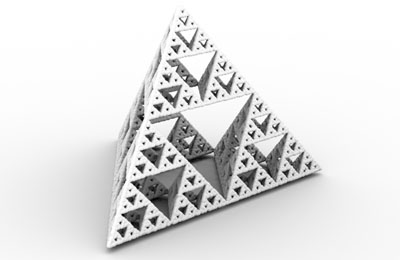Introduction

The python scripts presented in this tutorial generate Sierpinski gaskets rendered as RenderMan "Points" and "Blobby's". The python code was a direct port from the RSL code presented in the tutorial RSL: Sierpinski Point Clouds. The technique used by both tutorials is taken from,

Fractals for the Classroom
by Maletsky, Perciante and Yunker
ISBN: 0-387-97041-X

As shown below, the progressive build up of points in a Sierpinski gasket occurs randomly. A slightly modified version of the python script "sierpinski.py" (listing 1) was used to generate the 2D cloud of points. Instead of specifying four points, the modified script only provided three ie.

inputs = [ [0,0,1], [1,0,-1], [-1,0,-1] ] #, [0,1.5,-0.2] ]

Listing 1 (sierpinski.py)

 # A RenderMan procedural primitive that outputs a Sierpinski # gasket as a RenderMan Points (RiPoints) primitive. import math, random, sys # Returns a point midway between point "p1" and point "p2" def halfStep(p1, p2): x = (p2 - p1) / 2 y = (p2 - p1) / 2 z = (p2 - p1) / 2 result = [p1 + x, p1 + y, p1 + z] return result # Randomly chooses a point from a list of points def pickVert(listOfPnts): numPnts = len(listOfPnts) index = math.floor(random.random() * numPnts); return listOfPnts[int(index)] args = sys.stdin.readline() while args: arg = args.split() pixels = float(arg) # this is ignored numpnts = int(arg) # number of points to generate dia = float(arg) # the "width" of each point # The points define the tetrahedron that will bound # the sierpinski gasket. inputs = [ [0,0,1], [1,0,-1], [-1,0,-1], [0,1.5,-0.2] ] # An arbitary seed point playpnt = [0.0, 0.5, 0.0] print('Points "P" [\n') # Begin the "Points" primitive for n in range(numpnts): pnt = pickVert(inputs) playpnt = halfStep(playpnt, pnt) print('%1.3f %1.3f %1.3f\n' % (playpnt, playpnt, playpnt)) print('] "constantwidth" [%f]\n' % dia) sys.stdout.write('\377') sys.stdout.flush() args = sys.stdin.readline() # read the next set of inputs

Listing 2 is a rib file that uses the unmodified version of the sierpinski.py script to generate a 3D Sierpinski gasket - figure 2.

Listing 2 (sierpinski.rib)

 Option "searchpath" "shader" "@:../shaders" Option "searchpath" "texture" "@:../textures" Option "searchpath" "archive" "../archives" Hider "stochastic" "int sigma"  "float sigmablur" [1.0] Display "sierpinski" "framebuffer" "rgb" Format 427 240 1 Projection "perspective" "fov" 25 ShadingRate 1 Translate 0 0 5.396 Rotate -3.718 1 0 0 Rotate 21.801 0 1 0 Translate -0.0 -0.65 0.0 Scale 1 1 -1 Imager "background" "background" [1 1 1] WorldBegin TransformBegin Attribute "stochastic" "int sigma"  # On Windows omit "/usr/bin/" Procedural "RunProgram" ["/usr/bin/python PATH_TO/sierpinski.py" "100000 0.01"] [-2 2 -2 2 -2 2] # somewhat arbitary bounding box values! TransformEnd TransformBegin Scale 50 1 50 Polygon "P" [-0.5 0 -0.5 -0.5 0 0.5 0.5 0 0.5 0.5 0 -0.5] "st" [0 0 0 1 1 1 1 0] TransformEnd WorldEnd

Sierpinski Blobby

Listing 3 uses the 3D points it generates to define a RenderMan Blobby - figure 3. For other examples of how to generate other Blobby shapes procedurally refer to the tutorial RenderMan Procedural Primitives:BlobbiesFigure 3 - Blobby with 100,000 points

Listing 3 (sierpinski_blobby.py)

 # sierpinski_blobby.py import math, random, sys def halfStep(p1, p2): x = (p2 - p1)/2 y = (p2 - p1)/2 z = (p2 - p1)/2 result = [p1 + x, p1 + y, p1 + z] return result def pickVert(listOfPnts): numPnts = len(listOfPnts) index = math.floor(random.random() * numPnts); return listOfPnts[int(index)] args = sys.stdin.readline() while args: arg = args.split() pixels = float(arg) numBlobs = int(arg) scale = float(arg) random.seed(1) inputs = [ [0,0,1], [1,0,-1], [-1,0,-1], [0,1.5,-0.2] ] playpnt = [0.0, 0.5, 0.0] print('Blobby %d [\n' % numBlobs) for n in range(numBlobs): print('1001 %d\n' % (n * 16)) print('0 %d' % numBlobs) for n in range(numBlobs): print(' %d' % n) print(']\n[\n') row1 = '%1.3f 0 0 0 ' % scale row2 = '0 %1.3f 0 0 ' % scale row3 = '0 0 %1.3f 0 ' % scale for n in range(numBlobs): pnt = pickVert(inputs) playpnt = halfStep(playpnt, pnt) row4 = '%1.3f %1.3f %1.3f 1' % (playpnt, playpnt, playpnt) print('%s %s %s %s\n' % (row1, row2, row3, row4) ) print('] [""]\n') sys.stdout.write('\377') sys.stdout.flush() args = sys.stdin.readline() # read the next set of inputs

Explorations

Some interesting effects can be achieved by editing the halfStep function so that it can divide the distance between between two points by any value. For example,

def halfStep(p1, p2, div):
x = (p2 - p1)/div
y = (p2 - p1)/div
z = (p2 - p1)/div
result = [p1 + x, p1 + y, p1 + z]
return result

Figures 4 and 5 show what happens when the "div" parameter changes from 0.6 to 0.7.